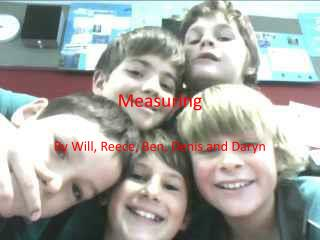Download PresentationMeasuring

# Measuring - PowerPoint PPT PresentationDownload Presentation## Measuring

- - - - - - - - - - - - - - - - - - - - - - - - - - - E N D - - - - - - - - - - - - - - - - - - - - - - - - - - -
##### Presentation Transcript

1. Measuring By Will, Reece, Ben, Denis and Daryn

2. LCD Screen • The size for the LCD screen is: • Length: 67cm • Width: 112cm • So the perimeter is 358cm • And the area is 0.75m2 and 750cm2

3. Classroom floor • The size of the classroom floor is: • Length:8m • Width:8m • So the perimeter of the classroom floor is 32m • The area is 64m2

4. A metal door leading into the classroom • The size of a door leading into the classroom is: • Length:205cm • Width:81cm • So the perimeter is 572cm • And the area is 1.66m2 and 166cm2

5. One Sliding Whiteboard • The size of one sliding whiteboard is: • Length:112cm • Width:136cm • So the perimeter is 496cm • And the area is 1.52m2 or 152cm2

6. Exercise Book • The size of an exercise book is: • Lenght:30cm • Width:21cm • So the perimeter is 1.02m • And the area is 6.30m

7. Rectangular table • The size of the rectangular table is: • Length: 74cm • Width: 150cm • So after adding it up it ends up to be 4.48m • And the area is 1.11m2 and 111cm2

8. A pigeon hole • The size of a pigeon hole is: • Length: 36cm • Width: 38cm • So the perimeter is 148cm • And the area is 1368cm2

9. A window • The size of the this window is: • Length:81cm • Width:81cm • So the perimeter is 324 • And the area is 6561cm2

10. Laptop • The size of this laptop is: • Length: 26cm • Width: 36cm • So the perimeter is 124cm • And the area is 936cm2

11. Paper Bin • The size of the paper bin is: • Length: 72cm • Width: 36cm • So the perimeter is 360 • And the area is 2592cm2

12. Reading Box • The size of the reading box is: • Length: 28 • Width: 17 • So the perimeter is 90cm • And the area is 476cm2

13. Book Shelf • The size of the book shelf is: • Length: 160cm • Width: 90cm • So the perimeter is 500 • And the area is 144cm2